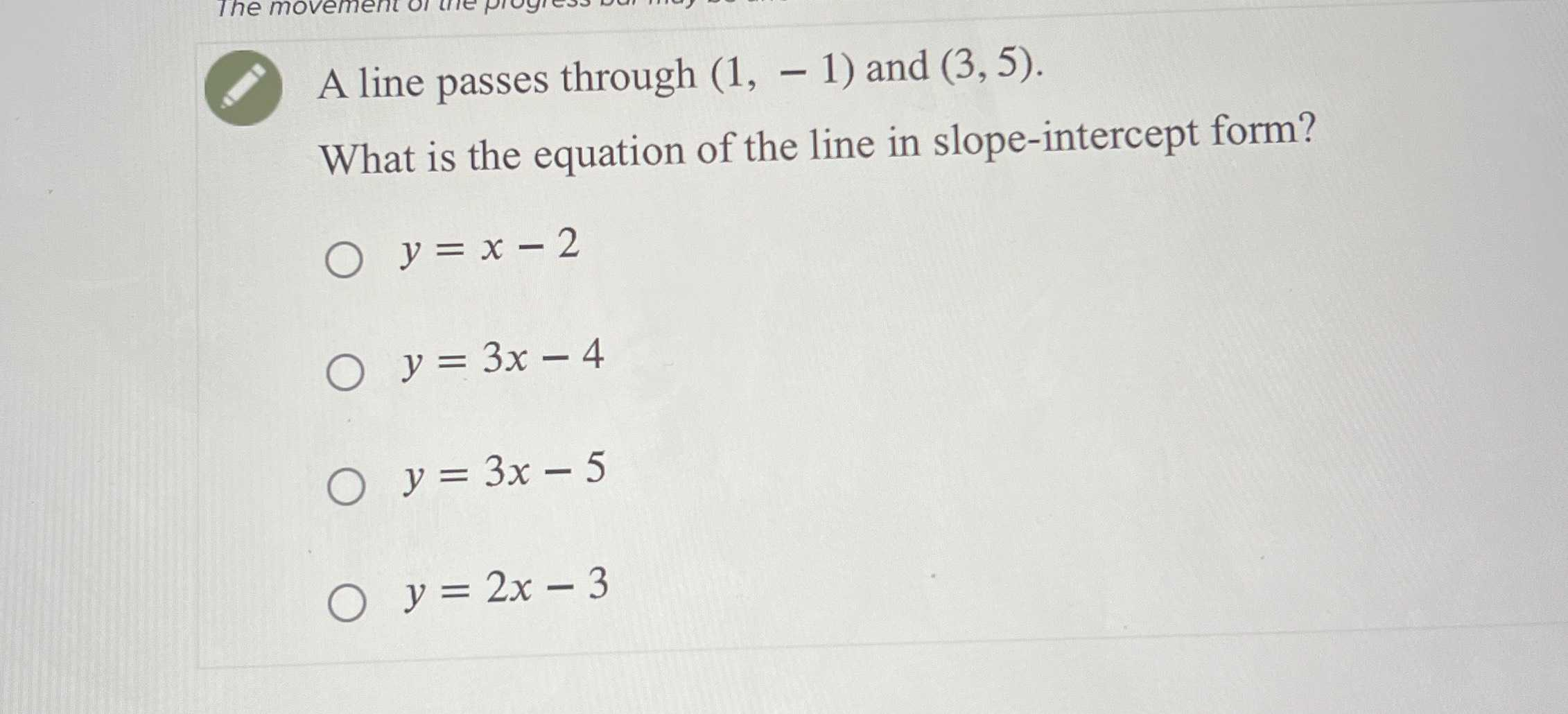### Still have math questions?

Algebra
QuestionA line passes through $$( 1 , - 1 )$$ and $$( 3,5 )$$

What is the equation of the line in slope-intercept form?

$$y = x - 2$$

$$y = 3 x - 4$$

$$y = 3 x - 5$$

$$y = 2 x - 3$$

$$y= 3x- 4$$